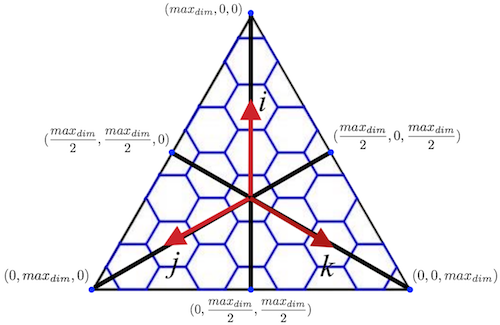Version: 4.x

# Determine the latitude/longitude coordinates of the center point of an H3Index cell

This operation is performed by function cellToLatLng. See the comments in the function source code for more detail.

The conversion is performed as a series of coordinate system conversions described below. See the page Coordinate Systems used by the H3 Core Library for more information on each of these coordinate systems.

1. The function _h3ToFaceIjk then converts the H3 index to the appropriate icosahedron face number and normalized ijk coordinate's on that face's coordinate system as follows:
• We start by assuming that the cell center point falls on the same icosahedron face as its base cell.
• It is possible that the cell center point lies on an adjacent face (termed an overage in the code), in which case we would need to use a projection centered on that adjacent face instead. We recall that normalized ijk coordinates have at most two non-zero components, and that in a face-centered Class II system the sum of those components is a resolution-specific constant value for cells that lie on the edge of that icosahedral face. We determine whether an overage exists by taking the sum of the ijk components, and if there is an overage the positive ijk components indicate which adjacent face the cell center lies on. A lookup operation is then performed to find the appropriate rotation and translation to transform the ijk coordinates into the adjacent face-centered ijk system.1. The face-centered ijk coordinates are then converted into corresponding Hex2d coordinates using the function _ijkToHex2d.
2. The function _hex2dToGeo takes the Hex2d coordinates and scales them into face-centered gnomonic coordinates, and then performs an inverse gnomonic projection to get the latitude/longitude coordinates.